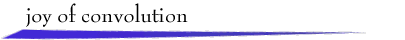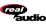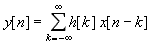Welcome!The behavior of a linear, time-invariant discrete-time system with input signalx[n] and output signal y[n] is described by the convolution sumThe signal h[n], assumed known, is the response of thesystem to a unit-pulse input.

The convolution summation has a simple graphical interpretation.First, plot h[k] and the "flipped and shifted" x[n - k]on the k axis, where n is fixed. Second, multiply thetwo signals to obtain a plot of the summand sequence indexed by k.Summing the values of this sequence with respect to kyields y[n]. These operations can be repeatedfor every value of n of interest.

To explore graphical convolution, selectsignals x[n] and h[n] from the provided examples below,or use the mouse to draw your own signal or to modify a selectedsignal. Then click at a desired value of n on the firstk axis. After a moment, h[k] and x[n - k] will appear. Drag the n symbol along the k axis to change thevalue of n. For each n, the corresponding summandh[k]x[n - k] and output value y[n] will bedisplayed in their respective windows.

Exercises.You need a Java-compatible browser to see the demo.return to demonstrations page

 Applet by Steve Crutchfield